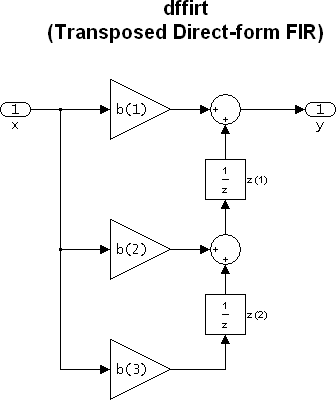# dfilt.dffirt

Discrete-time, direct-form FIR transposed filter

## Syntax

```Hd = dfilt.dffirt(b) Hd = dfilt.dffirt ```

## Description

`Hd = dfilt.dffirt(b)` returns a discrete-time, direct-form FIR transposed filter, `Hd`, with numerator coefficients `b`.

`Hd = dfilt.dffirt` returns a default, discrete-time, direct-form FIR transposed filter, `Hd`, with `b`=1. This filter passes the input through to the output unchanged.The resulting filter states column vector is

`$\left(\begin{array}{c}z\left(1\right)\\ z\left(2\right)\end{array}\right)$`

## Examples

Create a direct-form FIR transposed discrete-time filter with coefficients from a 30th order lowpass equiripple design:

```b = firpm(30,[0 .1 .2 .5]*2,[1 1 0 0]); Hd = dfilt.dffirt(b) ```